# Solid Shapes (polyhedrons, prisms) and Symmetry

Go back to  'Geometry'

## Solid Shapes

Solid shapes are three dimensional shapes who have length, breadth and height as the three dimensions.

Here are a few solid shapes.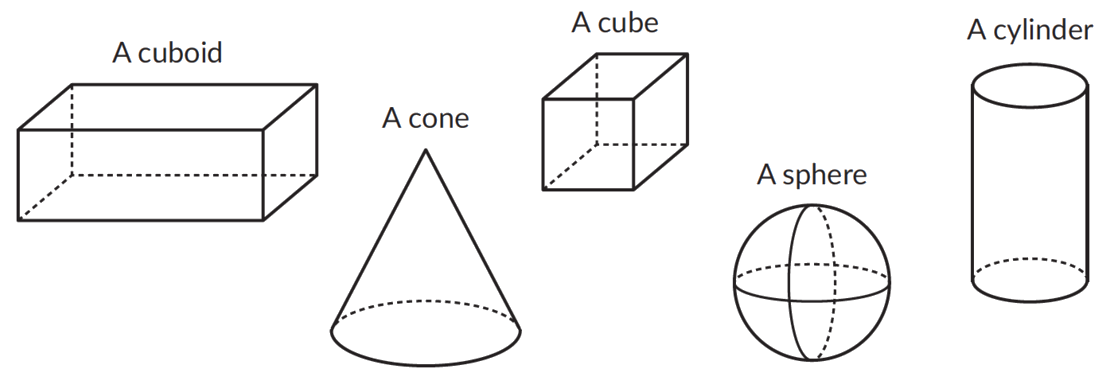### Solid shapes have faces, edges and vertices.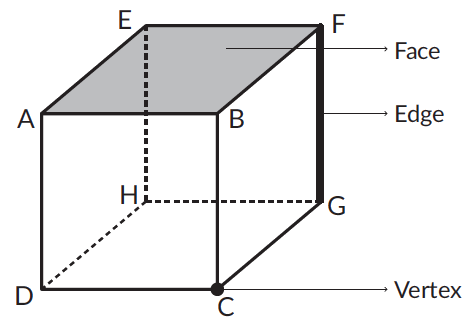## Polyhedron

A solid shape whose faces are all polygons is called a polyhedron.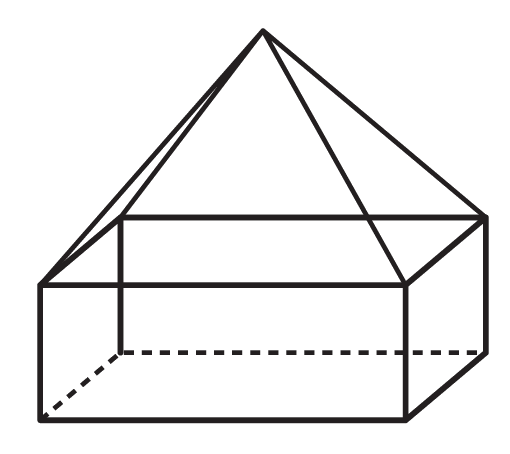### Pyramid

A pyramid is a polyhedron with: a polygon as its base and all other faces as triangles that meet at a point (Apex).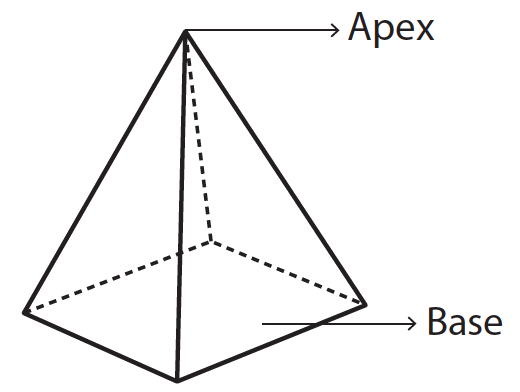Given below is a table showing the number of faces, edges, vertices for different polyhedrons.

 Solid Shapes Faces Edges Vertices Triangular pyramid (It's also called a Tetrahedron.)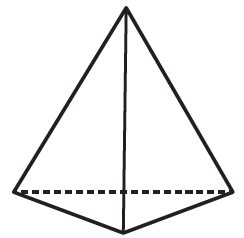4 6 4 Square Pyramid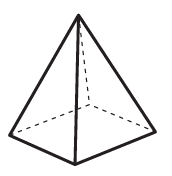5 8 5 Pentagonal Pyramid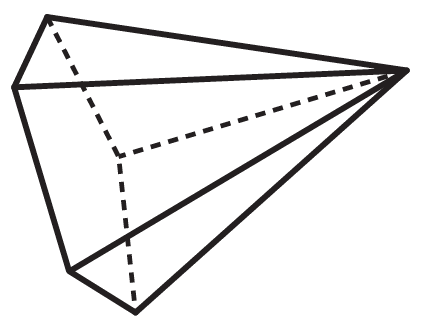6 10 6

### Prism

A prism is a polyhedron with two identical faces that are parallel and all other faces as parallelograms.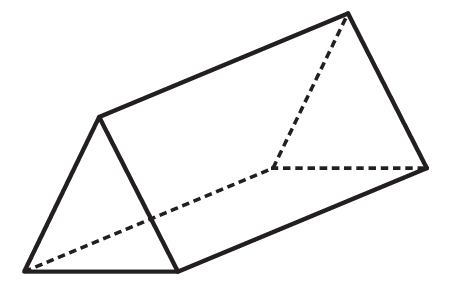Given below is a table showing the number of faces, edges, vertices for different types of prisms.

 Solid Shapes Faces Edges Vertices Triangular Prism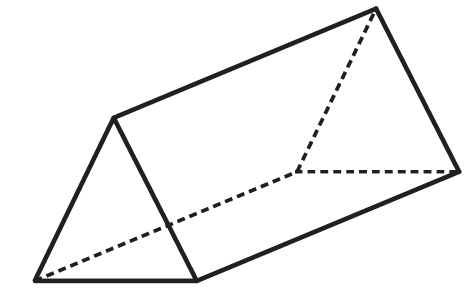5 9 6 Square Prism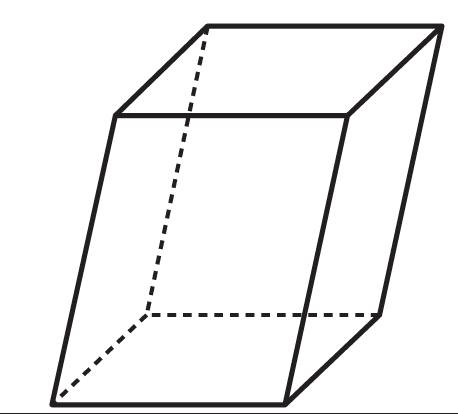6 12 8 Pentagonal Prism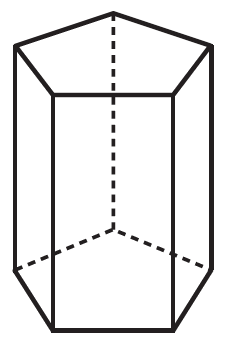7 15 10

### Formula - Euler’s formula

For every polyhedron, the value of $$F + V – E$$ is . This formula is called Euler’s formula

Let’s consider a few examples,

 Shape Faces Edges Vertices $$F + V – E$$5 8 5 5 + 5 - 8 = 2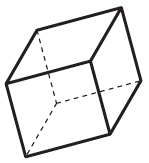6 12 8 6 + 8 - 12 = 2

## Symmetry

### What is a line of symmetry?

A figure is said to be symmetrical about a line if:

- the line divides the figure into two identical parts and
- one part exactly matches the other part when the figure is folded along that line.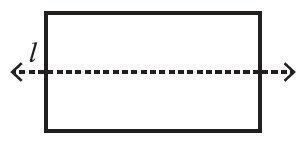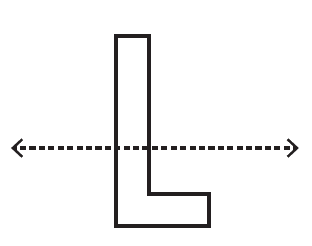Figure A                                                                   Figure B

In figure A the line of symmetry divides the figure into two identical parts. However, in figure B the line does not divide the figure into two identical parts.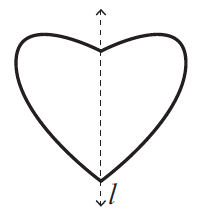This figure is symmetrical about the line $$l$$. This symmetry is called line symmetry.

A figure can have more than one line of symmetry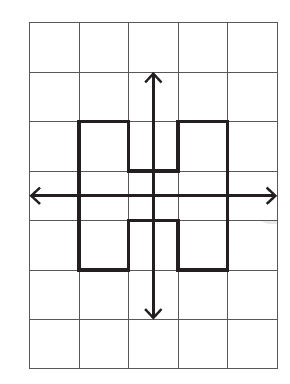### What is rotational symmetry?

A figure has rotational symmetry about a given point if it matches the original figure at least once before a complete rotation about that point.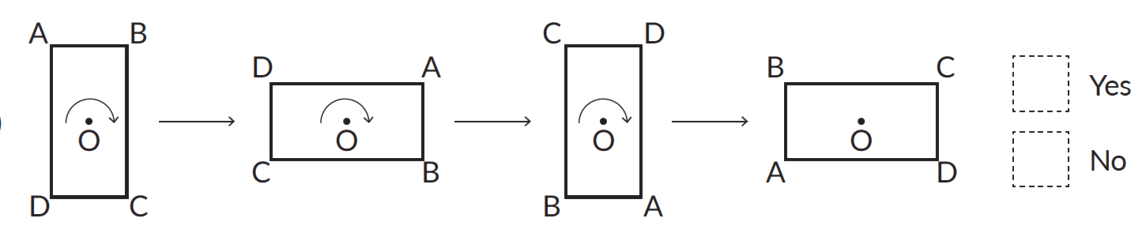The figure has rotational symmetry about ‘O’.

### What is the angle of rotation?

The angle by which an object is rotated is called the angle of rotation.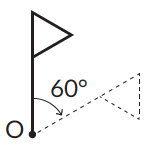For example, In the above given figure each dotted figure is obtained after rotating the given figure, the angle of rotation is 60°. A rotation of $$360^\circ$$ is called a complete rotation.

### What is order of symmetry?

The order of symmetry of a figure is the number of times the figure matches the original figure during a complete rotation $$(360^\circ).$$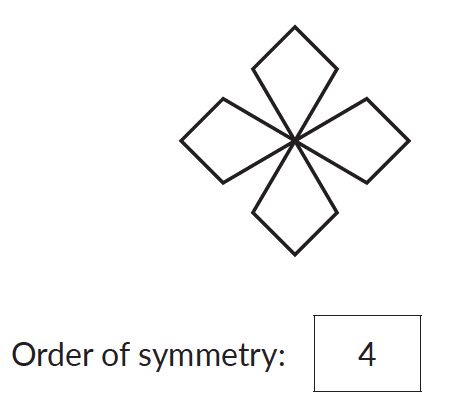### What is mirror symmetry?

A shape and its mirror image is always symmetrical about the mirror line.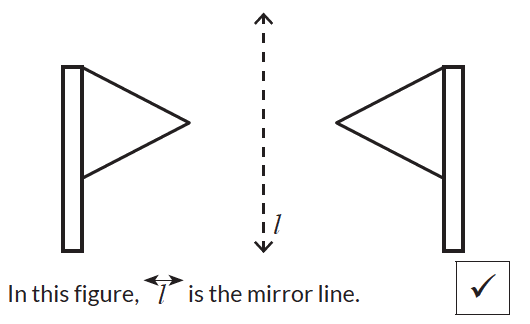In this figure, line L is the mirror line. The shape and its mirror image is symmetrical about the mirror line.

## Tips and tricks

• Tip: Make these shapes using plain sheet of paper. This would help visualise the solid shapes.
• Tip: Using the geosolid, move your finger along each shape to understand the concept of faces, edges and vertices.
• Tip: Use a paper that has grid lines on it. The grid lines make it easy to see if the line of symmetry is dividing the figure into two identical parts.
• Tip: Draw a free hand design on an A4 sheet. Now fold the paper in to one half. If the figure is exactly symmetrical than one part should match the other.

### Common mistakes or misconceptions

Misconception 1: A line of symmetry is always vertical.
A line of symmetry can be vertical, horizontal or diagonal as long as it divides the figure into two identical parts.

1. Which is a pentagonal pyramid?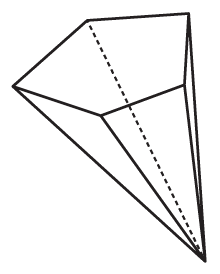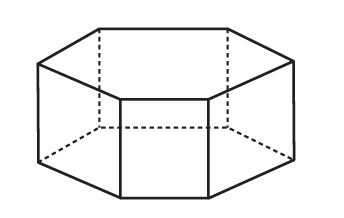2. Which is a pentagonal prism?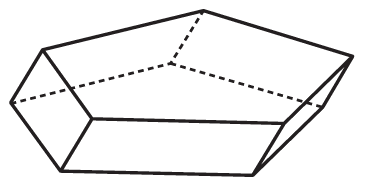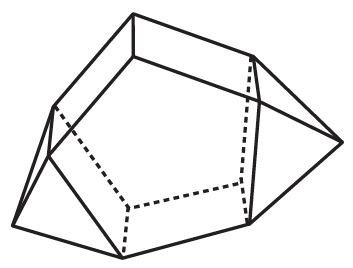3. Which is a hexagonal prism?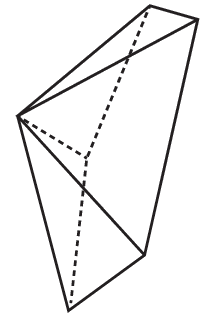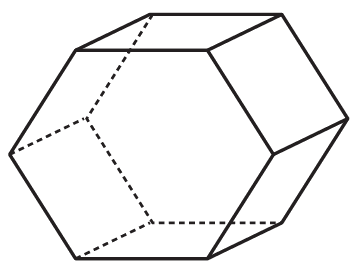4. Draw all the possible lines of symmetry for each letter of the English alphabet. Also write in the number of lines of symmetry.a. Number of lines of symmetry ________________________b. Number of lines of symmetry________________________c. Number of lines of symmetry________________________

5. Write yes or no if the first figure is the mirror image of the second figure, about the given line $$l.$$

a.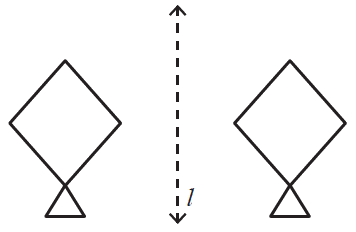b.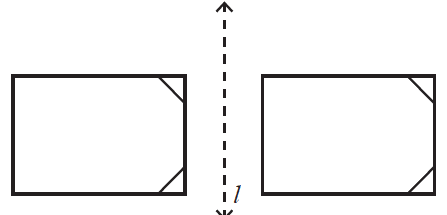Shapes and Symmetry
Shapes and Symmetry
Solid shapes
Solid shapes
Solid Shapes and Symmetry
Solid Shapes and Symmetry
Polygons and Solid Shapes